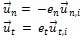# FINITE_MASS_BOUNDARY_CONDITION

Specifies the finite mass boundary conditions and interaction parameters for a particle surface.

AcuTrace Command

## Syntax

FINITE_MASS_BOUNDARY_CONDITION ("name") {parameters}

User-given name.

## Parameters

particle_surface or surface (string) [no default]
Name of the particle surface.
wall_type or type (enumerated) [=reflect]
Wall type.
reflect
Particles reflect at surface.
stop or trap
Particles stop at surface but remain active.
terminate or escape
Particles stop at surface and become inactive.
wall_en_type or en_type (enumerated) [=constant]
Type of normal coefficient of restitution. Applies only if wall_type is reflect.
constant
Coefficient is constant.
piecewise_linear or linear
Coefficient is a piecewise linear function of the normal component of the impact velocity.
cubic_spline or spline
Coefficient is a cubic spline function of the normal component of the impact velocity.
constant_wall_en or wall_en [=1.0]
Normal coefficient of restitution. Applies only if wall_type is reflect and wall_en_type is constant.
wall_en_curve_fit_values or en_values [no default]
A two-column array of normal-velocity/normal-coefficient-of-restitution data values. Used when wall_en_type is piecewise_linear or cubic_spline. Applies only if wall_type is reflect.
wall_et_type or et_type (enumerated) [=constant]
Type of tangential coefficient of restitution. Applies only if wall_type is reflect.
constant
Coefficient is constant.
piecewise_linear or linear
Coefficient is a piecewise linear function of the normal component of the impact velocity.
cubic_spline or spline
Coefficient is a cubic spline function of the normal component of the impact velocity.
constant_wall_et or wall_et [=1.0]
Tangential coefficient of restitution. Applies only if wall_type is reflect and wall_en_type is constant.
wall_et_curve_fit_values or et_values [no default]
A two-column array of normal-velocity/tangential-coefficient-of-restitution data values. Used when wall_et_type is piecewise_linear or cubic_spline. Applies if wall_type is reflect.

## Description

The FINITE_MASS_BOUNDARY_CONDITION command specifies the particle/wall interaction at a given AcuSolve particle surface of type wall, slip, or symmetry. An AcuSolve particle surface is a surface that is named either in a PARTICLE_SURFACE command or in a SIMPLE_BOUNDARY_CONDITION command.

For example, if either the command
PARTICLE_SURFACE( "upper_wall" ) {
type = wall
...
}
or the command
SIMPLE_BOUNDARY_CONDITION( "upper_wall" ) {
type = wall
...
}
appears in the AcuSolve input file for the AcuSolve run used by AcuTrace, upper_wall is an AcuSolve particle surface of type wall and the command
FINITE_MASS_BOUNDARY_CONDITION( "upper_wall" ) {
particle_surface = "upper_wall"
...
}
specifies the particle/wall boundary condition and interaction parameters at particle surface upper_wall. Moreover, the wall interaction parameters set in the FINITE_MASS command (wall_type, wall_en_type, constant_wall_en, wall_en_curve_fit_values, wall_et_type, constant_wall_et, and wall_et_curve_fit_values) are ignored for upper_wall. Only the parameters in the FINITE_MASS_BOUNDARY_CONDITION command, including any default parameter values, apply to particle surface upper_wall. Note in this example that any unique name can be used in the FINITE_MASS_BOUNDARY_CONDITION command, not just upper_wall; for example,
FINITE_MASS_BOUNDARY_CONDITION( "finite mass upper wall parameters" ) {
particle_surface = upper_wall
...
}
AcuTrace allows three different types of interaction when a particle hits a wall (here "wall" refers to a surface of type wall, slip, or symmetry):
• the particle reflects off the wall (wall_type = reflect)
• the particle stops but continues to be actively involved in the particle trace (wall_type = stop)
• the particle trace terminates (wall_type = terminate)

When a particle reflects off a wall, the normal and tangential components of its velocity, ${\stackrel{\to }{u}}_{n}$ and ${\stackrel{\to }{u}}_{t}$ (in the wall frame of reference) are given by

(1)where ${e}_{n}$ and ${e}_{t}$ are the normal and tangential coefficients of restitution, and ${\stackrel{\to }{u}}_{n,i}$ and ${\stackrel{\to }{u}}_{t,i}$ are the incident values of the normal and tangential components of the particle velocity. ${e}_{n}$ and ${e}_{t}$ always lie between 0 and 1.

Each coefficient can be specified as a constant, a piecewise linear function of the magnitude of the incident normal velocity, or a cubic spline function of the magnitude of the incident normal velocity. Note that AcuTrace clips the values of the coefficients so that they lie between 0 and 1.

In the first example below, the normal and tangential coefficients of restitution have constant values of 1.0:
wall_en_type        = constant
wall_et_type        = constant
wall_en             = 1.0
wall_et             = 1.0
In the next example, the normal and tangential coefficients of restitution have values of .1, .5, and .9 for incident normal velocity magnitudes of 1, 10, and 100, respectively. Linear interpolation is used for velocity magnitudes between 1 and 100; constant extrapolation is used for magnitudes less than 1 or greater than 100:
wall_en_type        = piecewise_linear
wall_et_type        = piecewise_linear
en_values           = { 1.0, 0.1 ;
10.0, 0.5 ;
100.0, 1.0 }
et_values           = { 1.0, 0.1 ;
10.0, 0.5 ;
100.0, 1.0 }
wall_en_type        = cubic_spline
wall_et_type         = cubic_spline